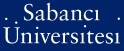# On algebraic curves in prime characteristic

Anbar, Nurdagül (2012) On algebraic curves in prime characteristic. [Thesis]PDF - Requires a PDF viewer such as GSview, Xpdf or Adobe Acrobat Reader361Kb

## Abstract

In this thesis we consider two problems related to algebraic curves in prime characteristic. In the first part, we study curves defined over the finite field Fq. We prove that for each sufficiently large integer g there exists a curve of genus g with prescribed number of degree r points for r = 1,..., m. This leads to the existence of a curve whose L-polynomial has prescribed coefficients up to some degree. In the second part, we consider curves defined over algebraically closed fields K of odd characteristic. We show that a plane smooth curve which has a K-automorphism group of order larger than 3(2g2 + g)([square root]8g + 1 + 3) must be birationally equivalent to a Hermitian curve.

Item Type: Thesis Artin-schreier extension. -- Sutomorphism. -- Curve. -- Degree r point. -- Function field. -- Hurwitz genus formula. -- Order sequence. -- Artin-Schreier genişlemesi. -- Otomorfizma. -- Eğri. -- Derecesi r olan nokta. -- Fonksiyonel cisimler. -- Hurwitz cins formülü. -- Derece dizisi. Q Science > QA Mathematics 26551 IC-Cataloging 20 Dec 2014 13:56 25 Mar 2019 17:11

Repository Staff Only: item control page